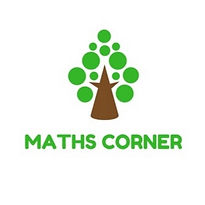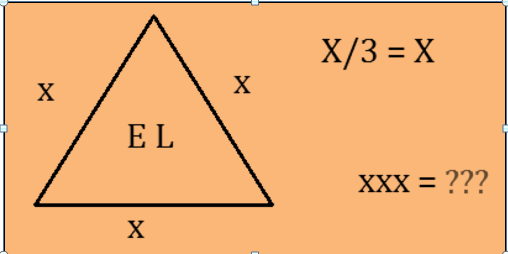MATHS CORNER [ 2018-19]

In order to provide opportunities for mathematical exploration and thinking Indian school Salalah Mathematics Department started Maths Corner. First and last week of every month mathematical questions and puzzles will display on it. All students can participate in it. Within two days students can hand over answers to the respective maths teachers. Name of the students those answered correctly will display on Maths corner.

The following are the questions and puzzles which have displayed on maths corner.

Q2. By using the numbers 8, 5, 1 and 1 exactly once and with the help of basic operation            (+, -, /, x), Can you form an expression with a value of 10?Q3.  In this 4 x 4 array each symbol stands for a different number. The sum of the                           symbols in three of the rows and three of the columns is given. What are the two                   missing totals?

###### ​Q4. Based on the picture below, what is the value of xxx =?Q7.    If 3 is the least prime factor of a number and 5 is             the least prime factor of another number. Find the           least prime factor of the sum of these numbers.               Give reason.

Q8.        IF          10 – 05 = 1

36 – 06 = 5

49 – 07 = 6

THEN    81 – 09 = ?

Q9. FIND THE VALUES OF A, B, C and D FROM THE FOLLOWING SUM:

(Each letter represents a different nonzero digit)

A    B    C    D

+       A    B     C

3    1    A     A

Q10. Find the missing number.Q11. CAN YOU SOLVE IT?

Q1. Ramu’s present age is one sixth of his father’s age. If we add the ages of Ramu, his           mother and father, the sum will be equal to 70. If Ramu’s father’s age become twice           that of Ramu’s age, the sum of the ages of Ramu, his mother and father will become         142. What is the present age of Ramu’s father?